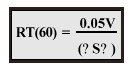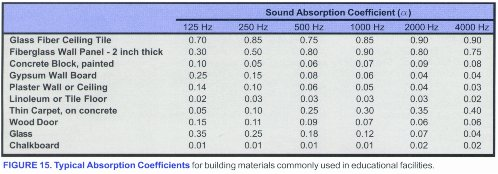## Reverberation Time for Gymnasiums and Multi-Purpose Rooms

The below excerpt from a booklet on classroom acoustics published by the Acoustical Society of America can help understand reverberation time for community and industrial applications.  Although this was written about classrooms, the principle is the same for all indoor spaces.

Source: Acoustical Society of America, A publication of the technical committee on architectural acoustics of the Acoustical Society of America.  https://asa.aip.org/classroom/booklet.html

Over 100 years ago, a Harvard physics professor named Wallace Clement Sabine developed the first equation for reverberation time, which has since been named after him and is still used today.  Reverberation time is defined as the length of time required for sound to decay 60 dB from its initial level.  Sabine’s simple formula iswhere:

RT (60) = reverberation time (seconds)

V = room volume (cubic feet)

S = surface area (square feet)

? = absorption coefficient of material(s) at given frequency

? indicates the summation of S times ? for all room surfaces

To use this formula, the volume of the room, surface area of each material in the room, and absorption coefficients for those materials must be known.  Absorption coefficients are measured in specialized laboratories, and represent the fraction of sound energy (not sound level – dB) the material will absorb as a decimal from 0 to 1.  Figure 15 gives absorption coefficients for common classroom materials.A commonly used one-number rating called NRC, noise reduction coefficient, is simply the average of the absorption coefficients at 250, 500, 1000, and 2000 Hz.  This simple, one-number rating can be useful for comparing the relative absorption of two materials; however, examining absorption coefficients in each octave band gives a better idea of the performance of a material at various frequencies.

Reverberation time is often calculated with the room unoccupied.  Since people and their clothing provide additional sound absorption, an unoccupied room is the worst-case scenario, though not an unreasonable one, since occupancy of most classrooms varies.  In a complete analysis, this calculation should be performed for each octave band, as the RT can vary widely at different frequencies.  However, for a quick estimate, the RT of a classroom can be calculated for just one octave band representative of speech frequencies, such as 1000 Hz.  If this RT is acceptable, then the RT throughout the speech range will likely be acceptable.